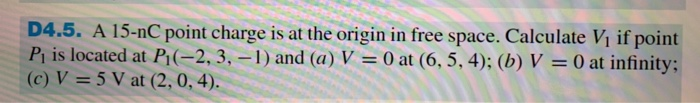### Create an Account

Home / Questions / D4.5. A 15-nC point charge is at the origin in free space. Calculate V1 if point P1 is located at P-

# D4.5. A 15-nC point charge is at the origin in free space. Calculate V1 if point P1 is located at P-D4.5. A 15-nC point charge is at the origin in free space. Calculate V1 if point P1 is located at P-2,3, --1) and (a) V = 0 at (6, 5, 4); (b) V = 0 at infinity; (c) V = 5 V at (2, 0, 4).

Apr 01 2020 View more View LessSubscribe To Get Solution